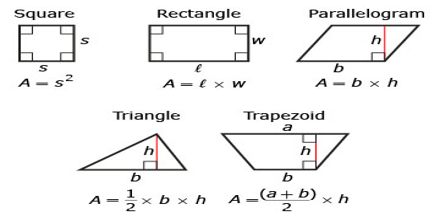Mathematic

# Area FormulasBasic objective of this lecture is to present on Area Formulas. It is a tutorial based lecture, describe all formulas related Area: Rectangle, Triangle, Parallelogram, Rhombus, Trapezoid and Kite. Area is measured in “square” units. The area of a figure is the number of squares required to cover it completely, like tiles on a floor. Area of a square = side times side. Since each side of a square is the same, it can simply be the length of one side squared.